### Home > CALC > Chapter 7 > Lesson 7.3.3 > Problem7-137

7-137.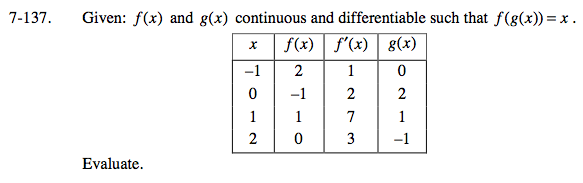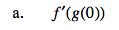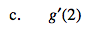Since g(2) = −1,
the inverse, f(−1) = 2.
Now f '(−1) = 1, so g '(2) = __
Refer to the hint in part (b)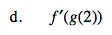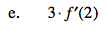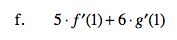Refer to the hint in part (b).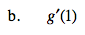How to find derivative of inverse functions:
A function, f, and its inverse, g, will have reciprocal derivatives at corresponding (x, y)→(y, x) values.

$\text{So, if }f\text{ has coordinate point (1, 2) and at }x=1, \text{ its derivative is }\frac{3}{5}.$

$\text{Then }g\text{ has coordinate point (2, 1) and at }x=2, \text{its derivative is }\frac{5}{3}.$